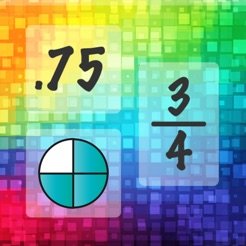Screenshots

•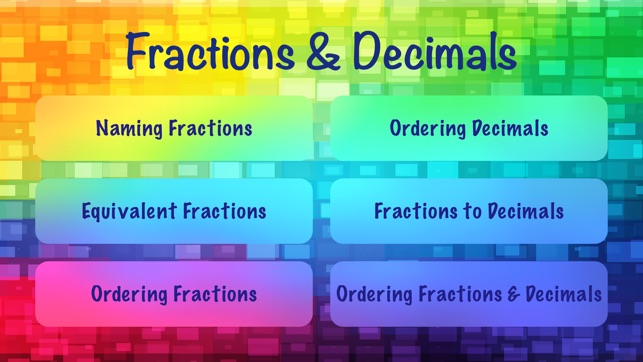•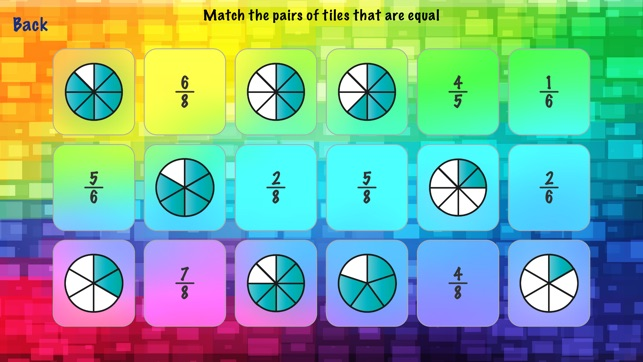•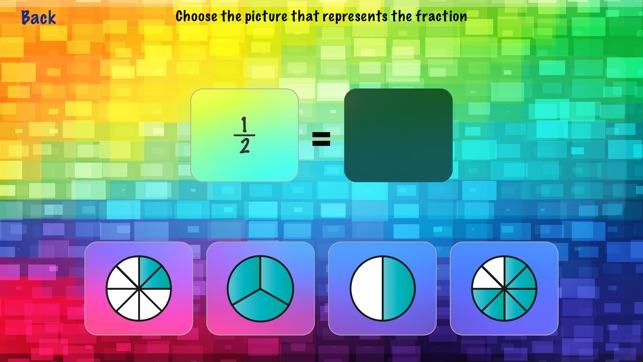•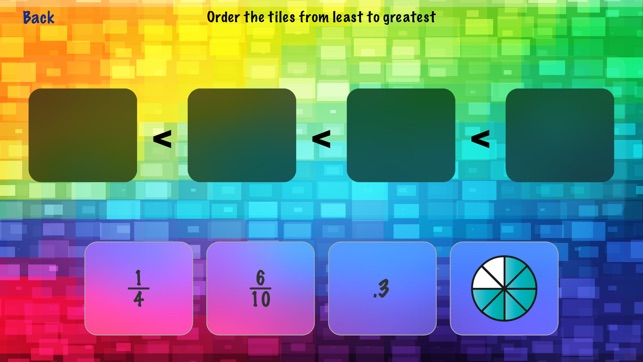•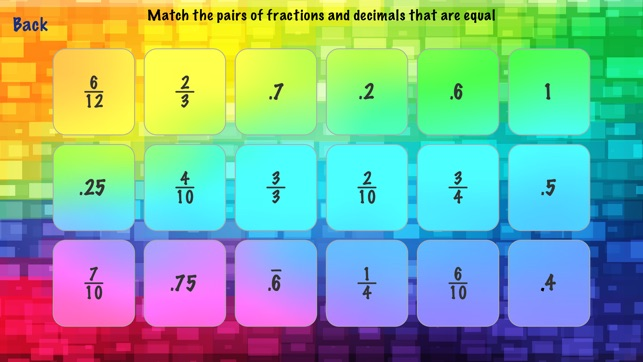Description

Fractions & Decimals has fun and simple activities to practice a range of fraction and decimal concepts such as: naming fractions, equivalent fractions, ordering fractions and decimals, and equivalent fractions and decimals.

The app has the following activities:

Naming Fractions:
Multiple Choice Mode: Choose the picture that represents the fraction
Matching Mode: Match fractions to the pictures of the fractions

Equivalent Fractions:
Multiple Choice Mode: Choose the fraction equivalent to the given fraction
Matching Mode: Match the pairs of equivalent fractions

Ordering Fractions:
Order fractions from least to greatest (2 levels)

Ordering Decimals:
Order decimals from least to greatest

Fractions to Decimals:
Multiple Choice Mode: Choose the decimal that is equal to the given fraction (2 levels)
Matching Mode: Match the pairs of fractions and decimals that are equal to each other. (2 levels)

Ordering Fractions and Decimals:
Order the fractions, decimals and their picture representations in order from least to greatest (2 levels)

Information

Provider
TicTapTech, LLC
Size
5.8 MB
Category
Education
Compatibility

Requires iOS 8.1 or later. Compatible with iPhone, iPad and iPod touch.

Languages

English

Age Rating
Rated 4+
© 2015 TicTapTech LLC
Price
₹ 79

Supports

•